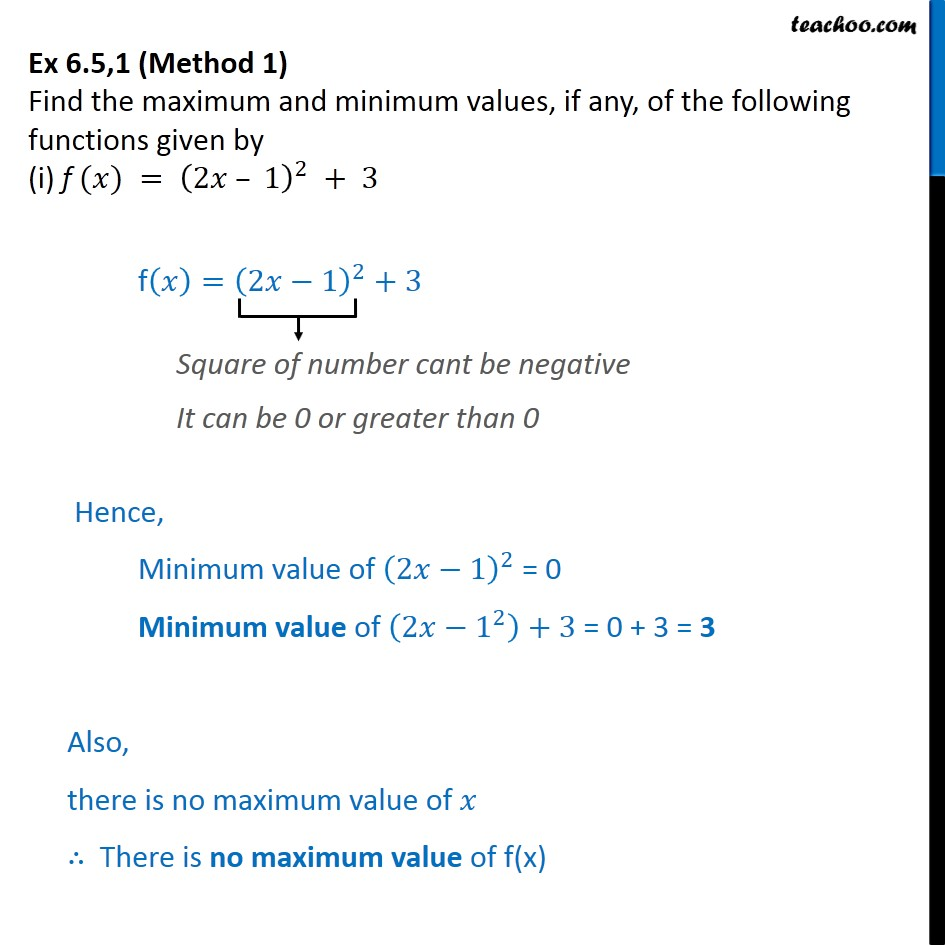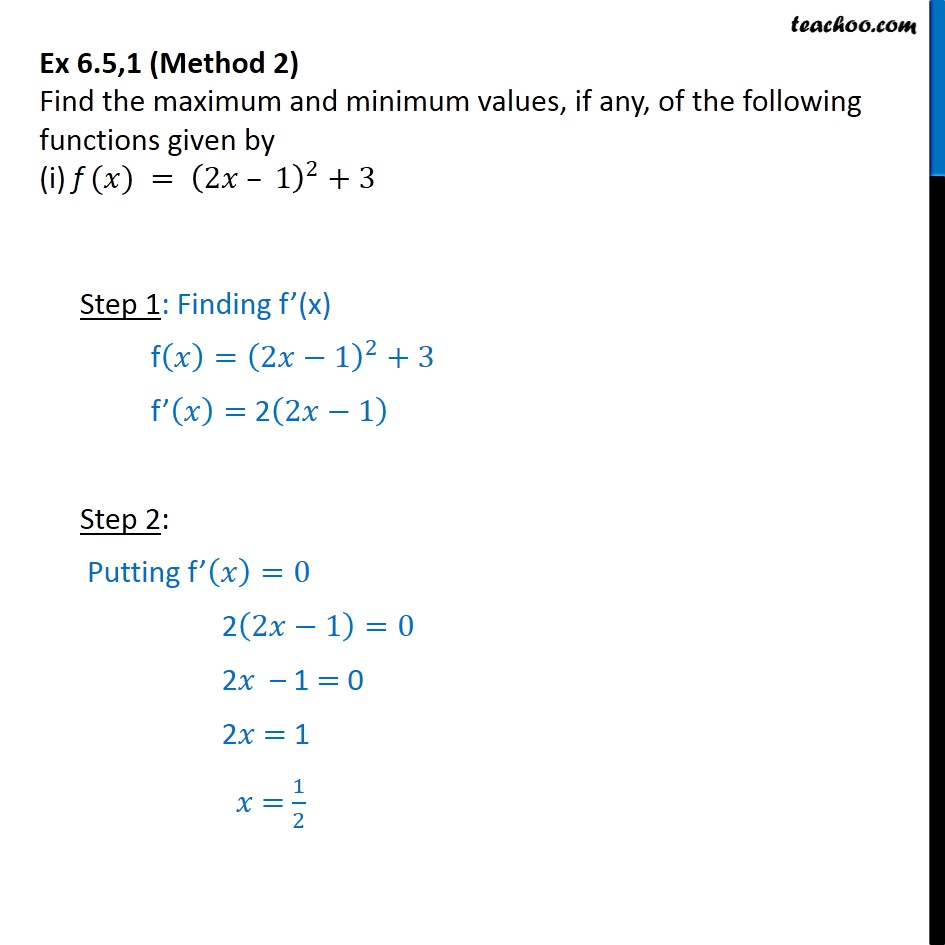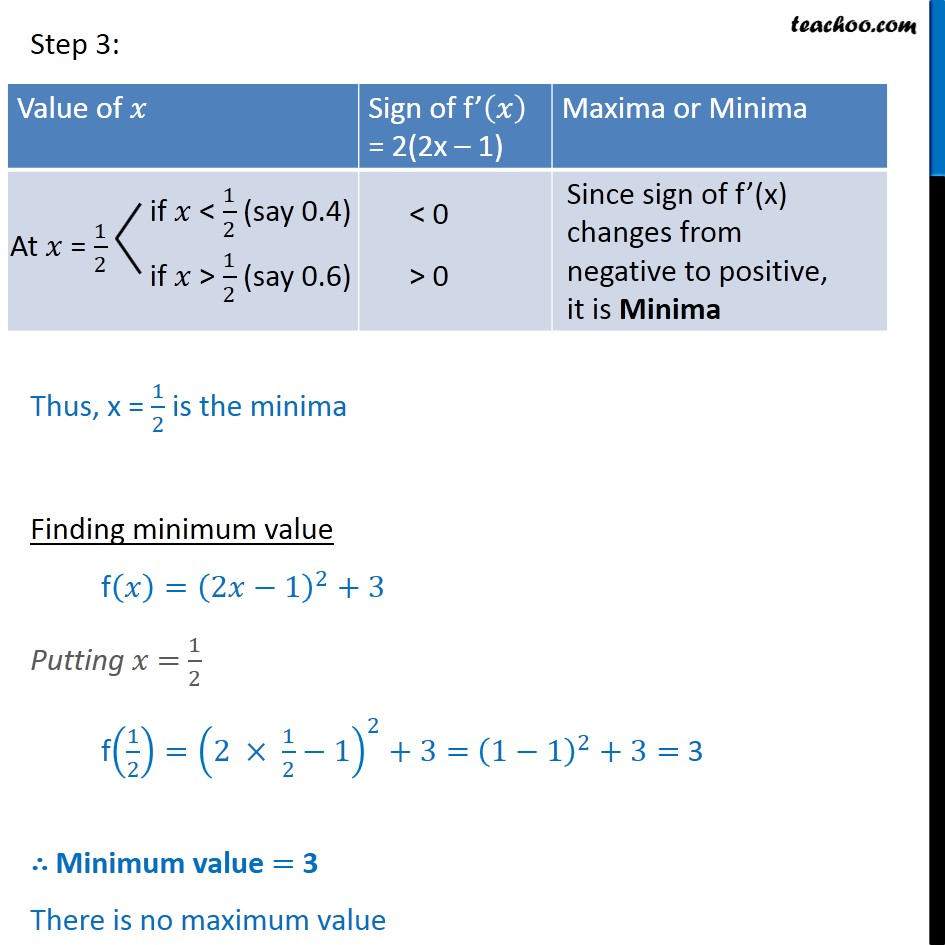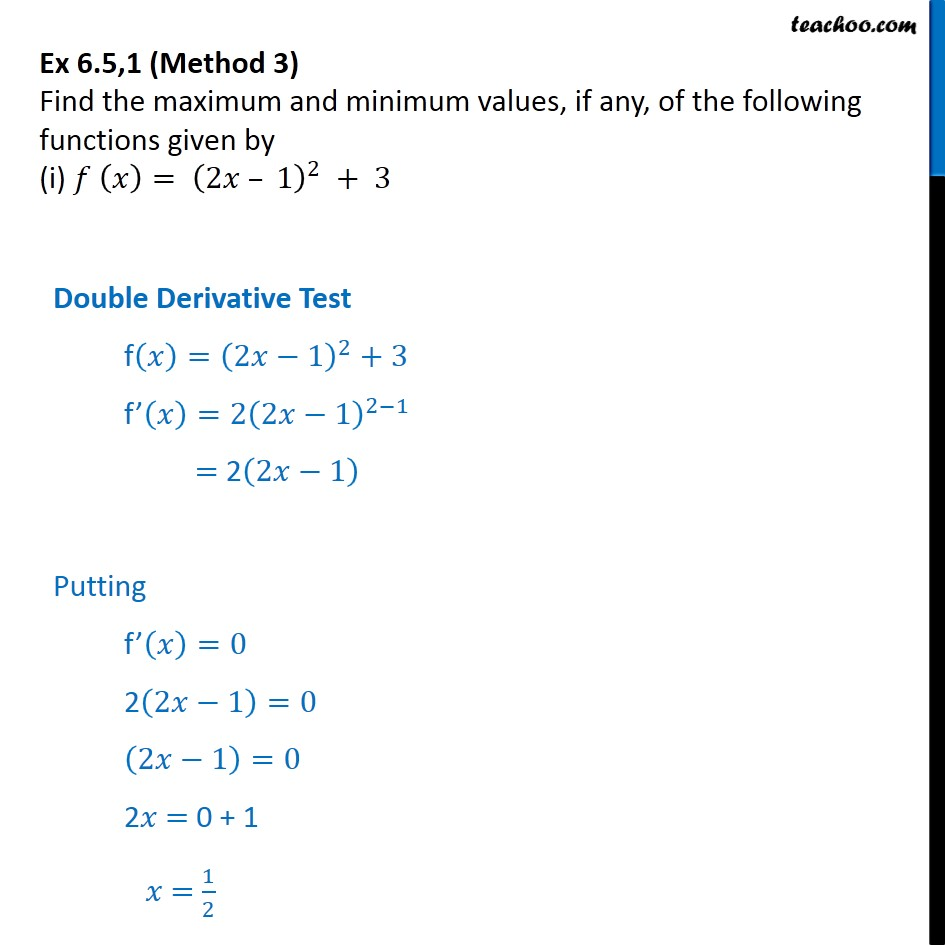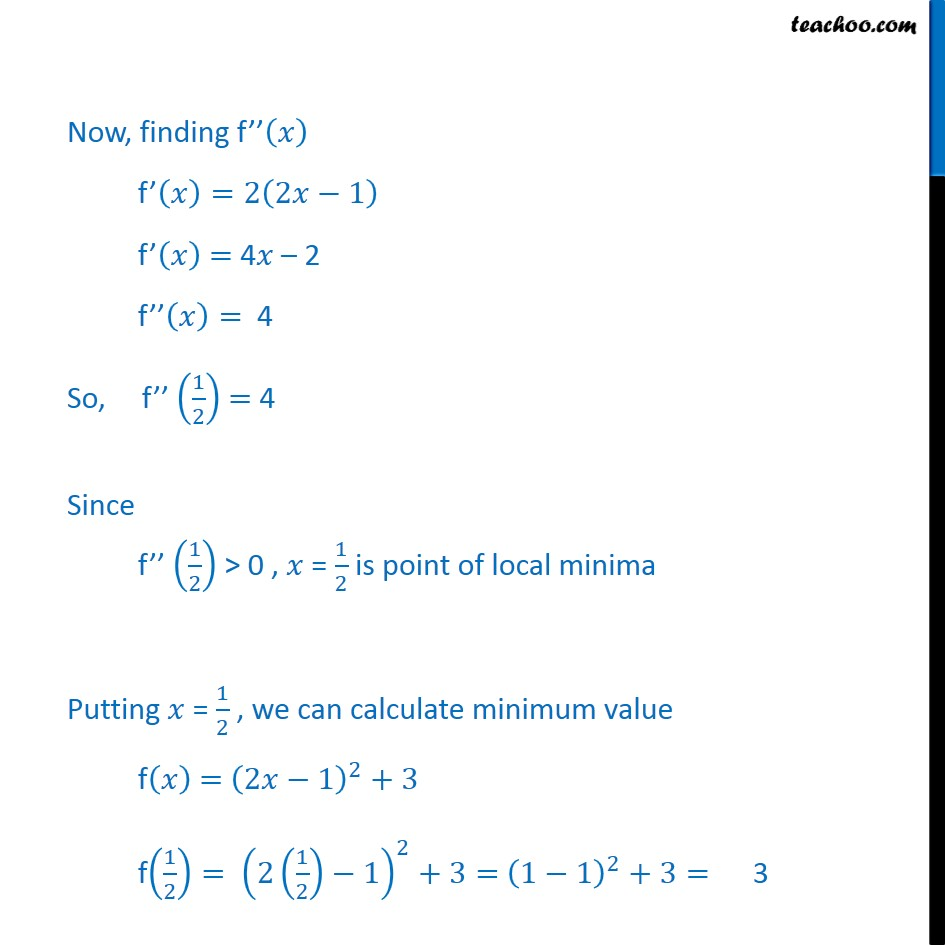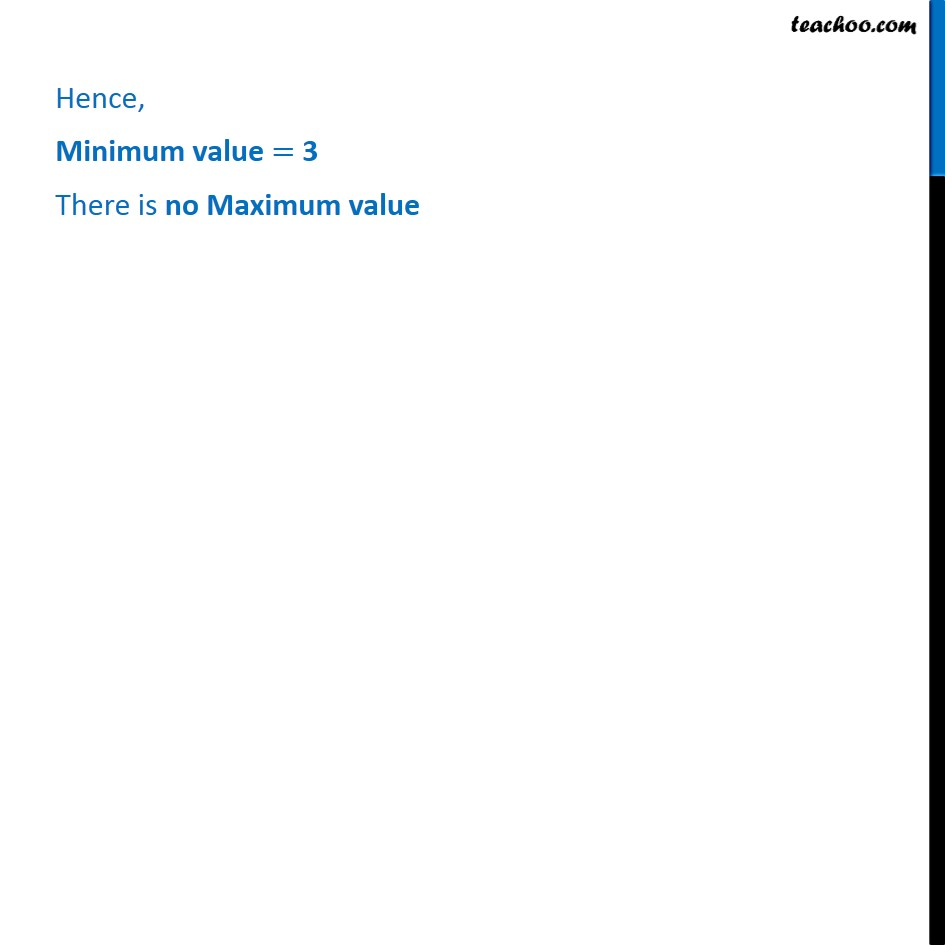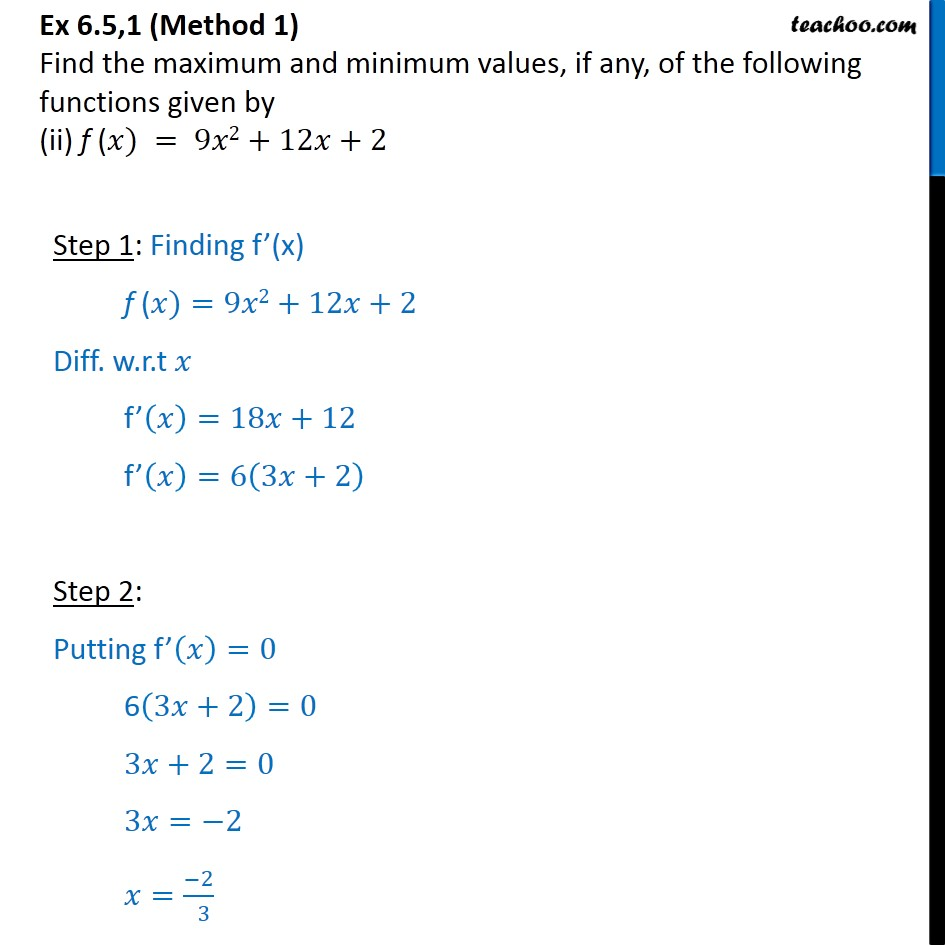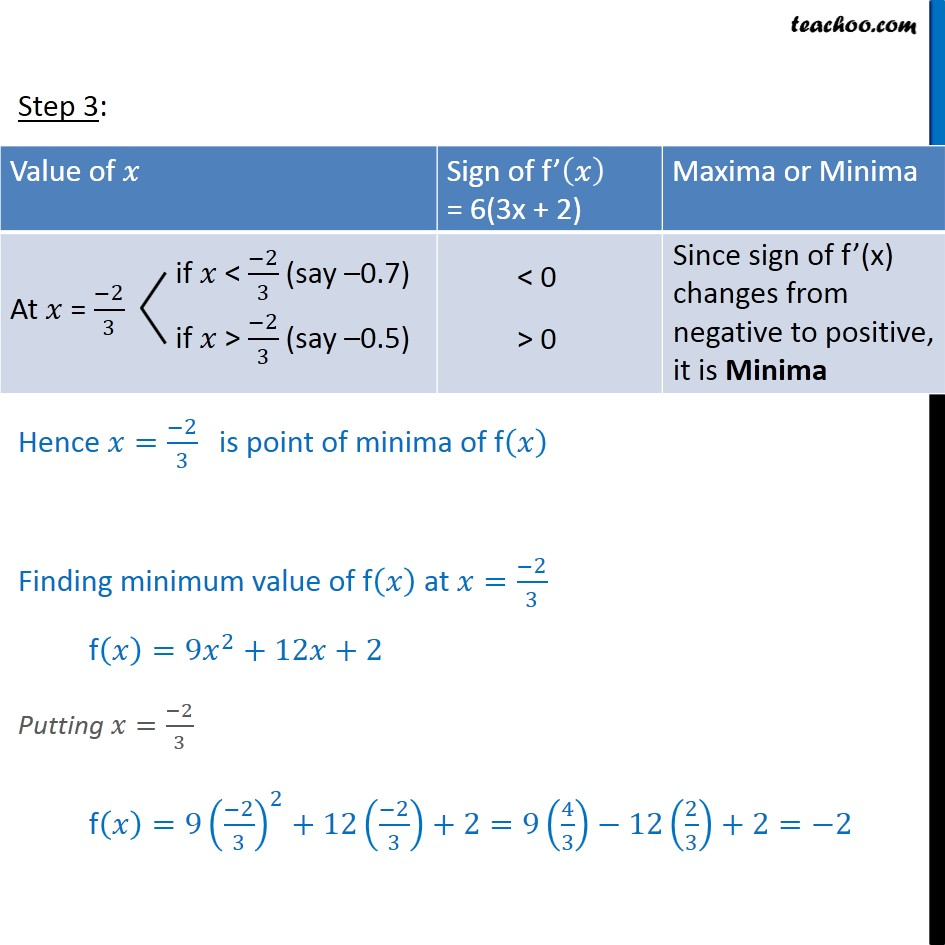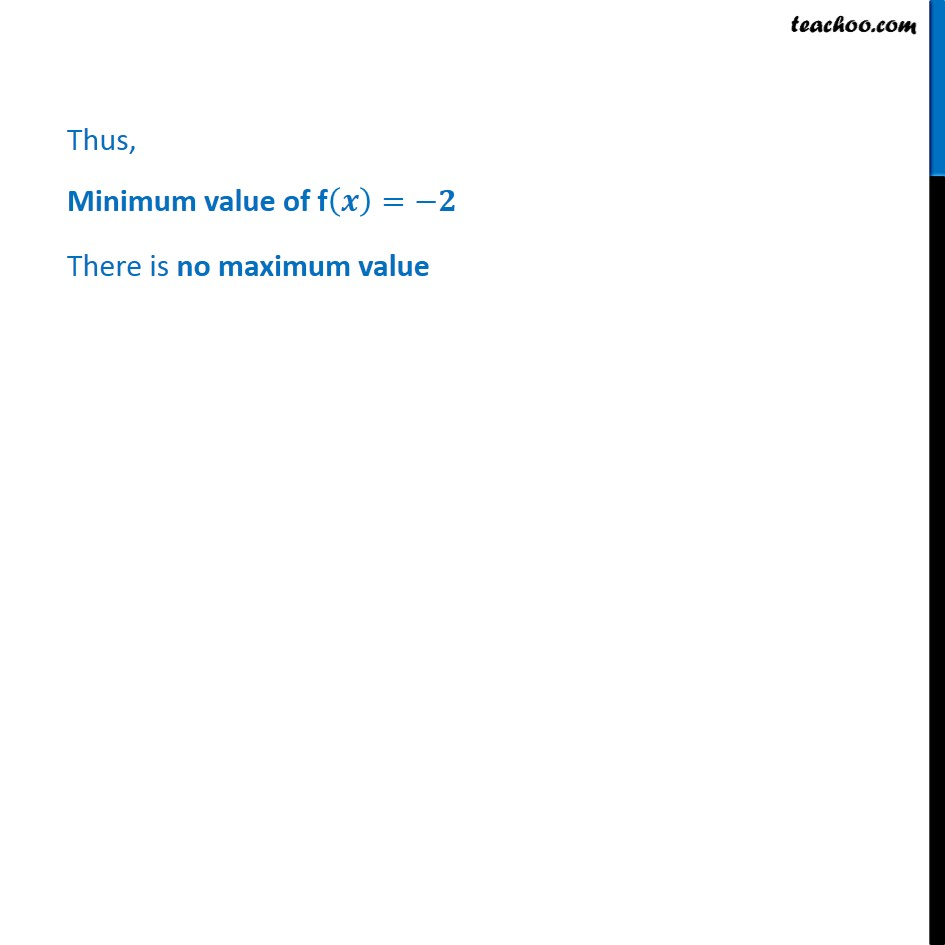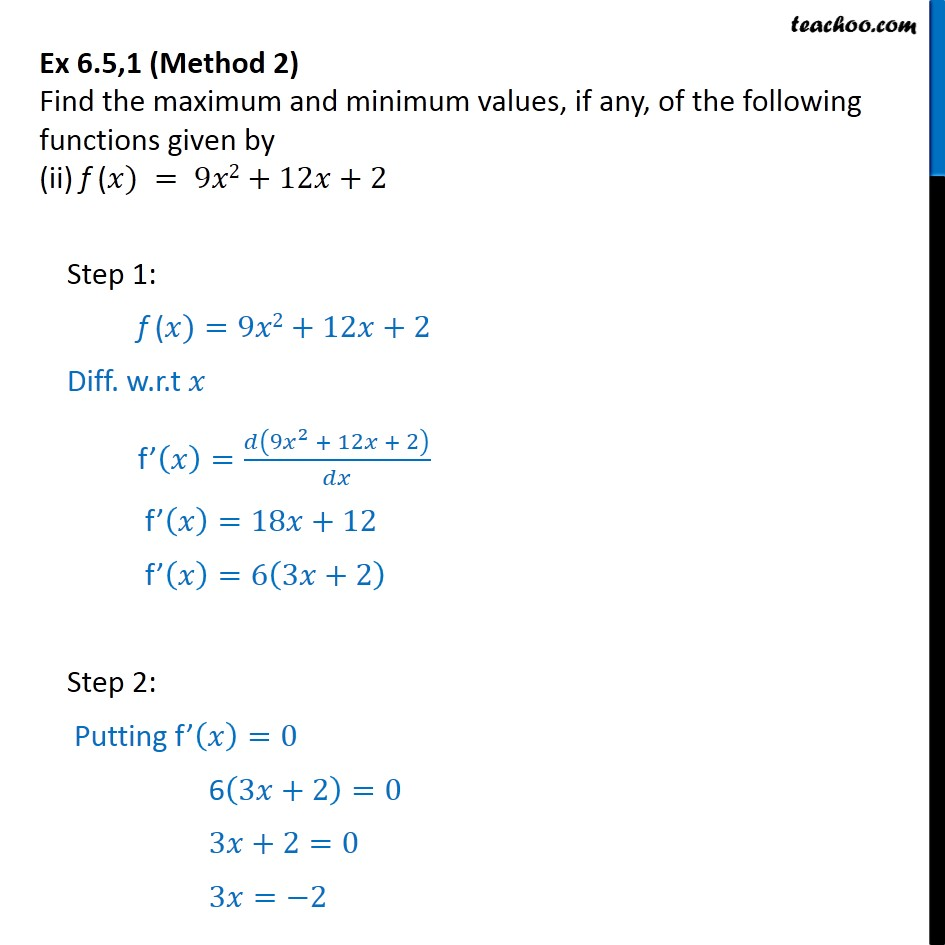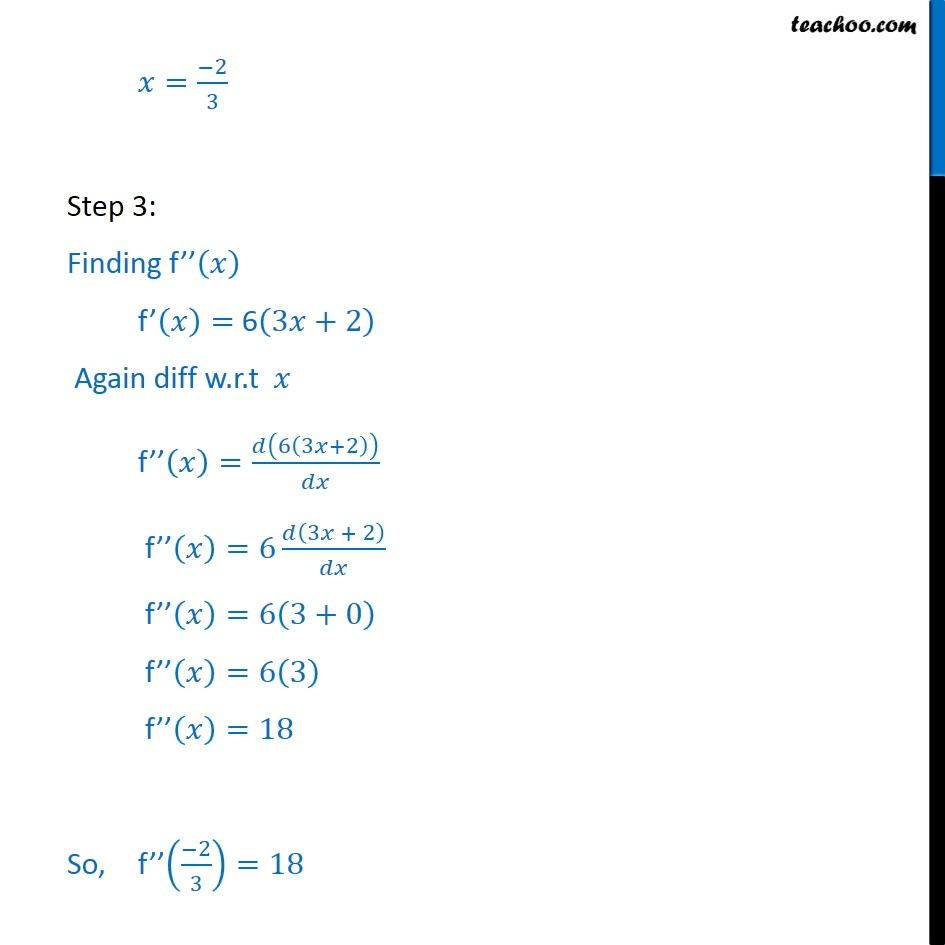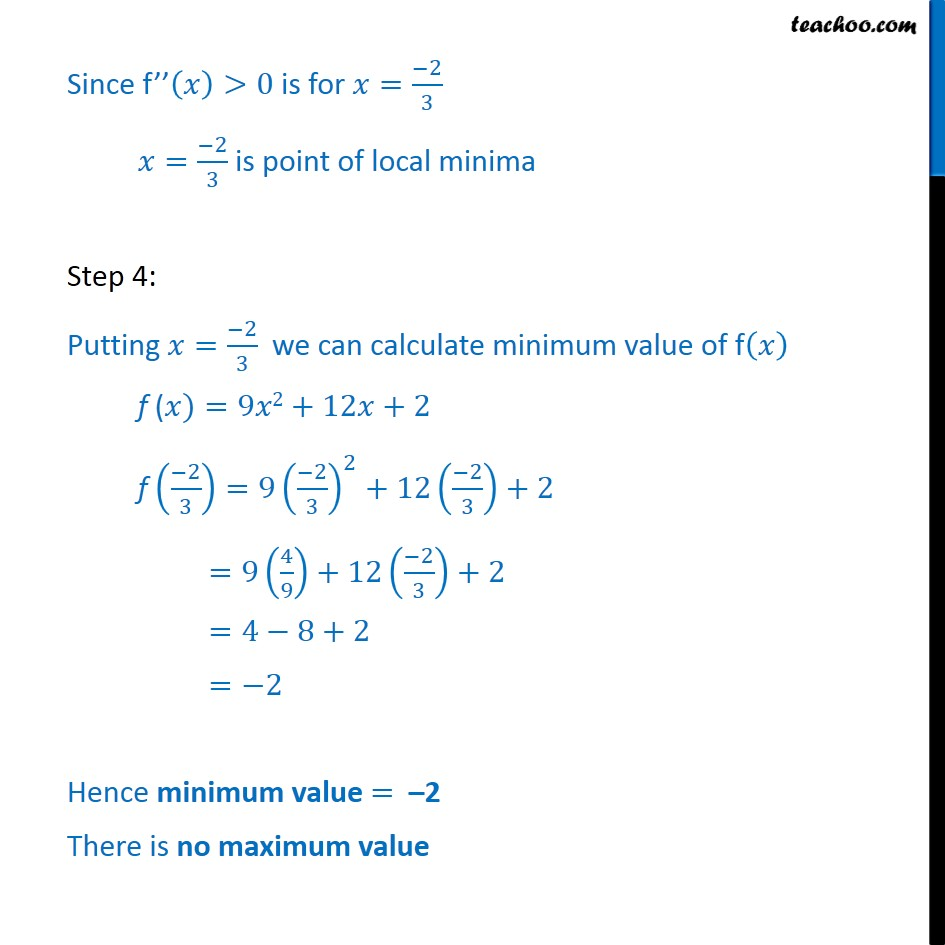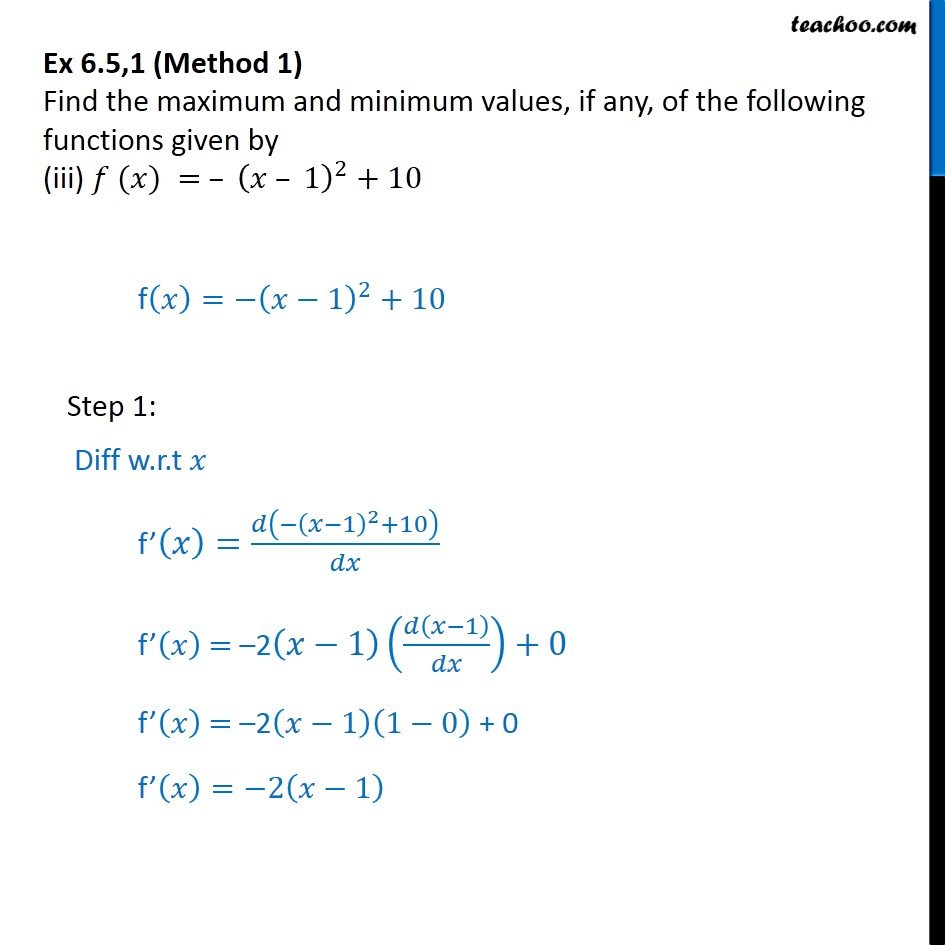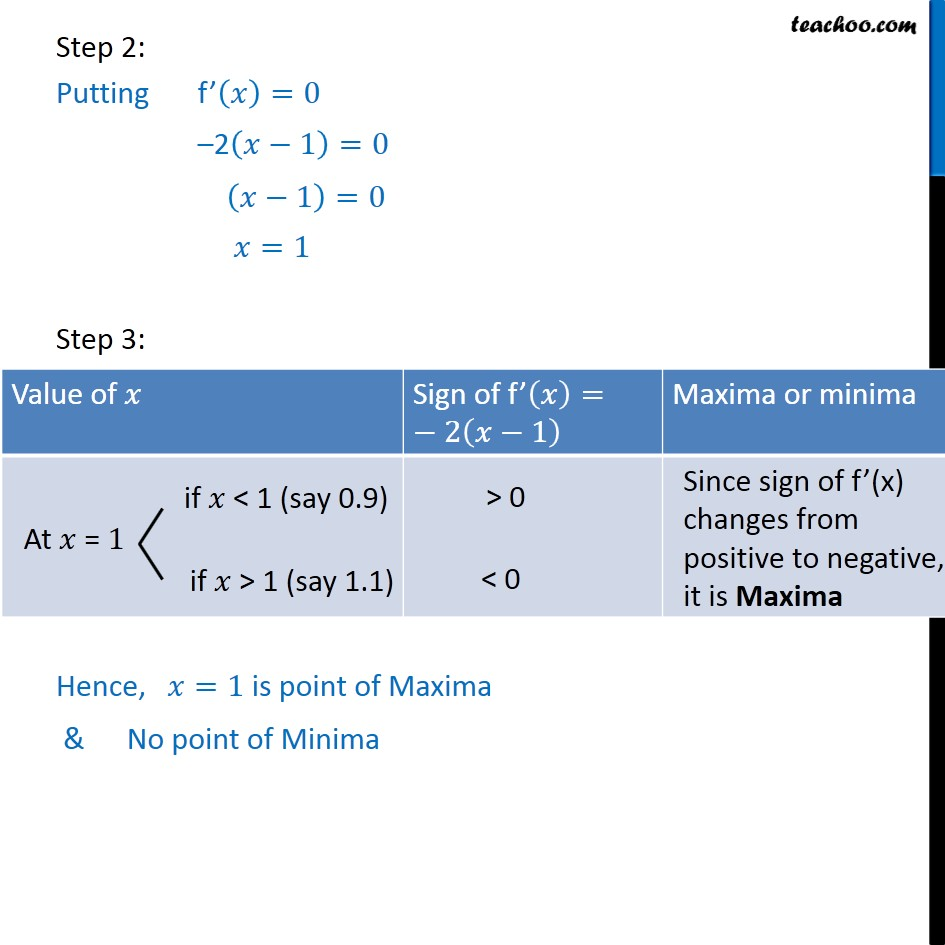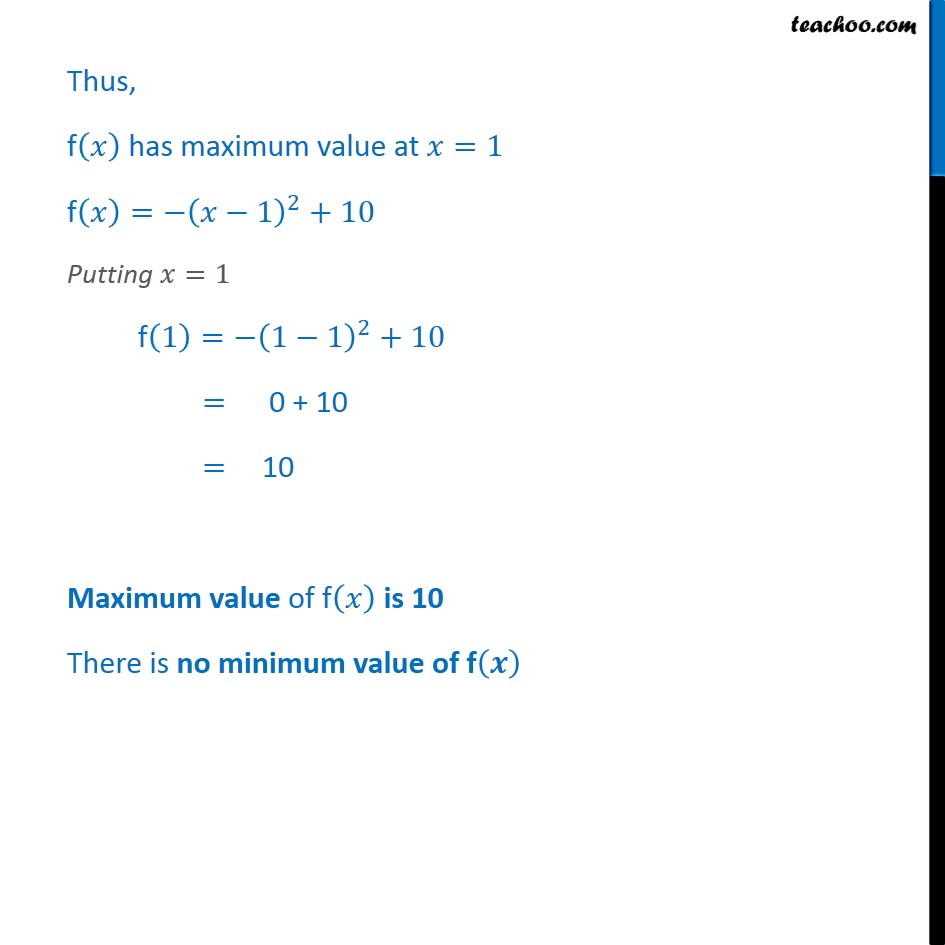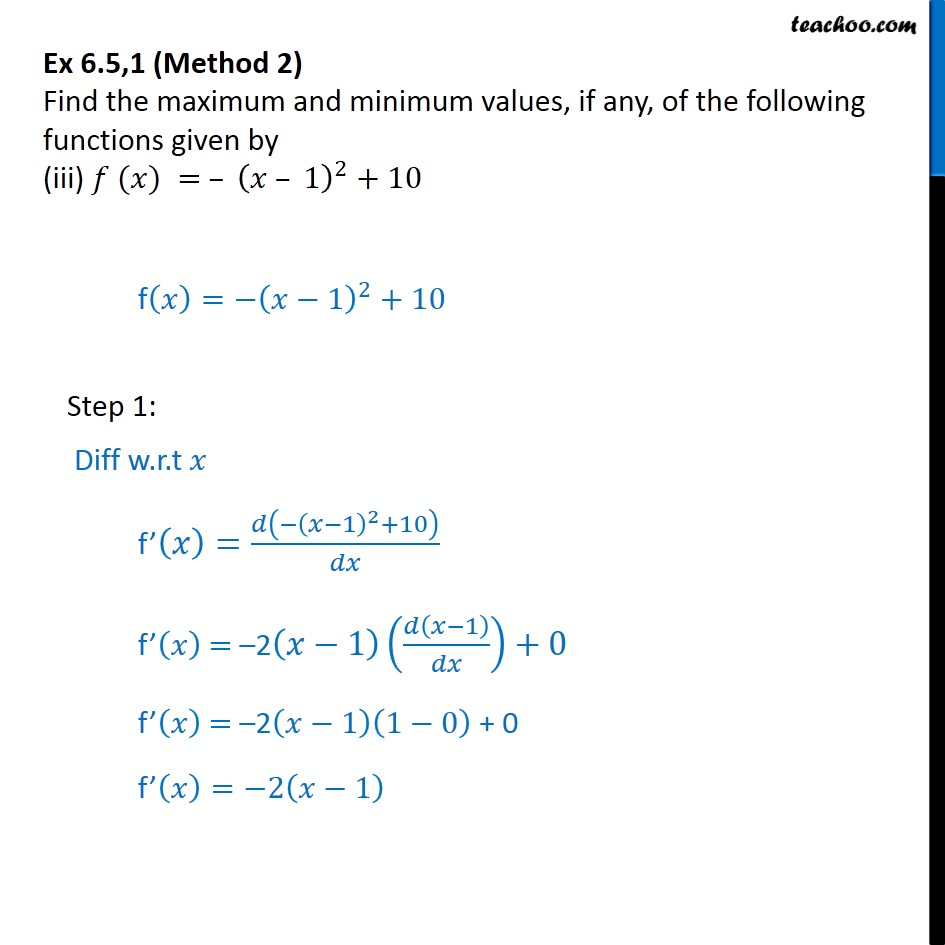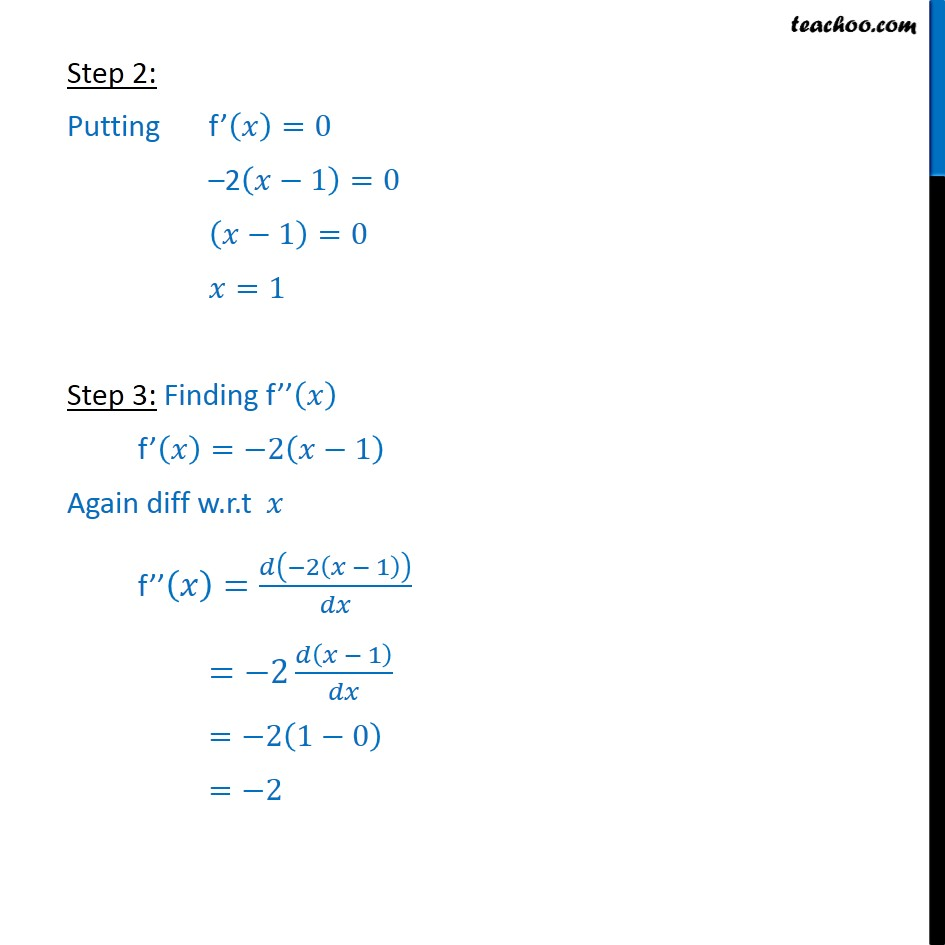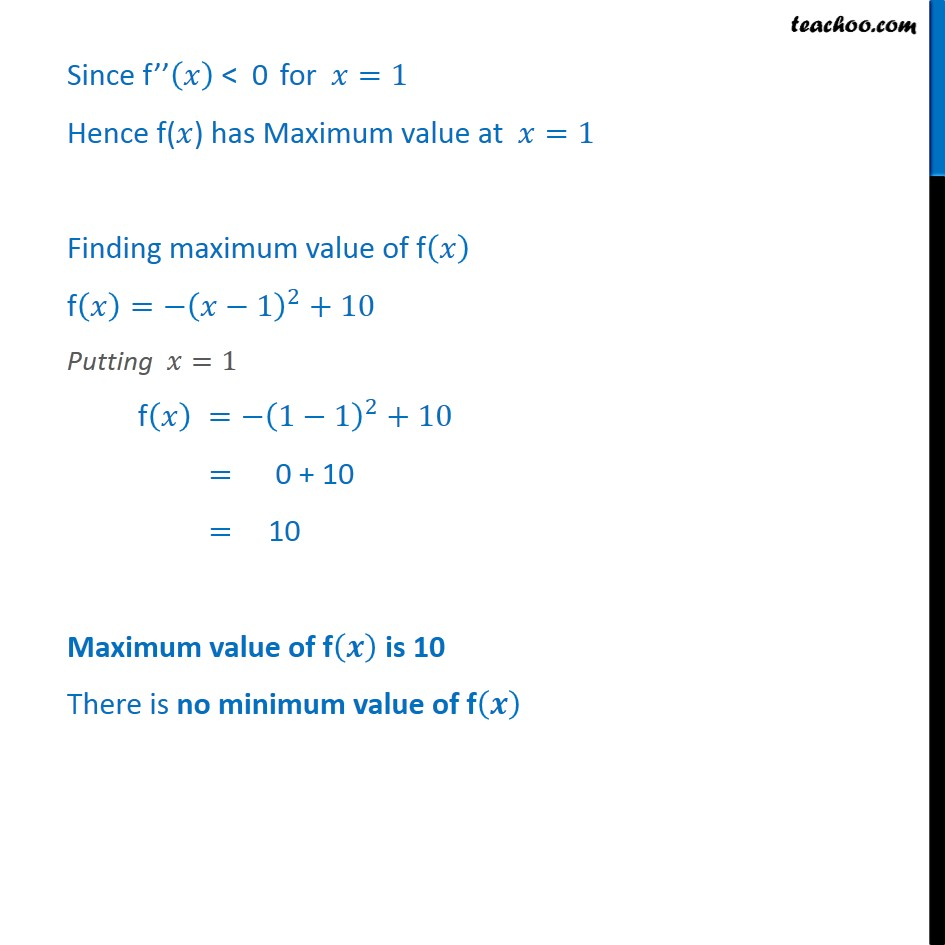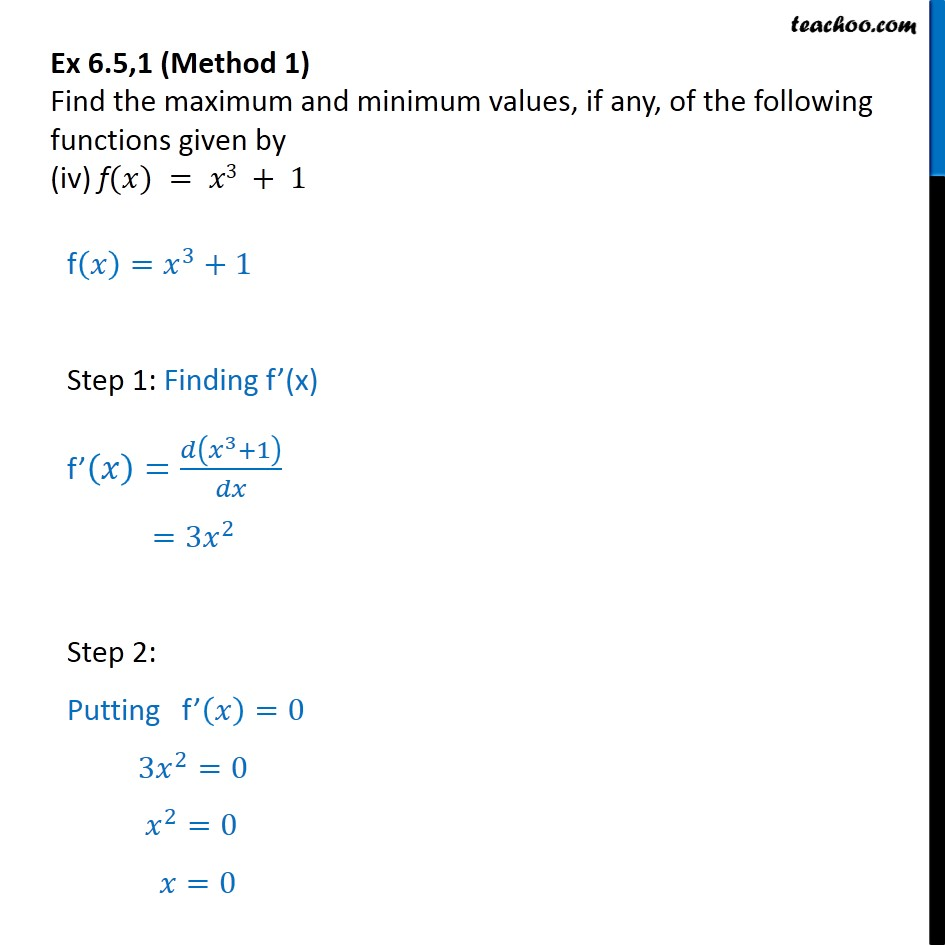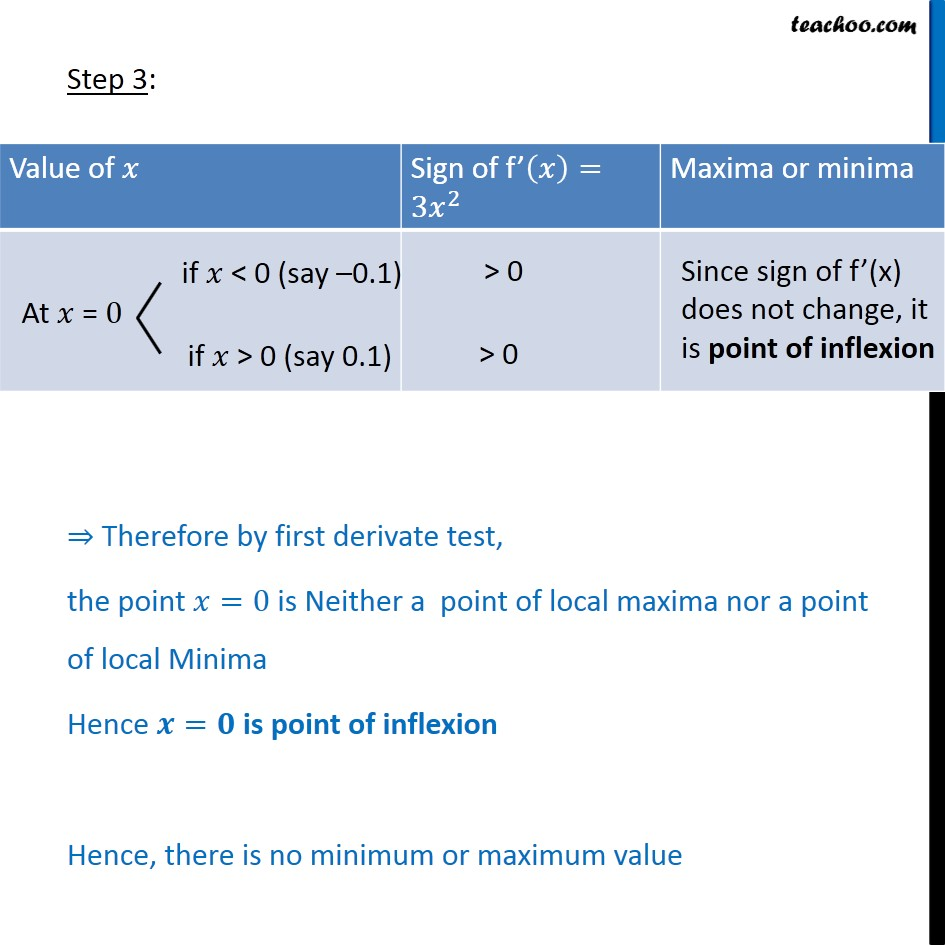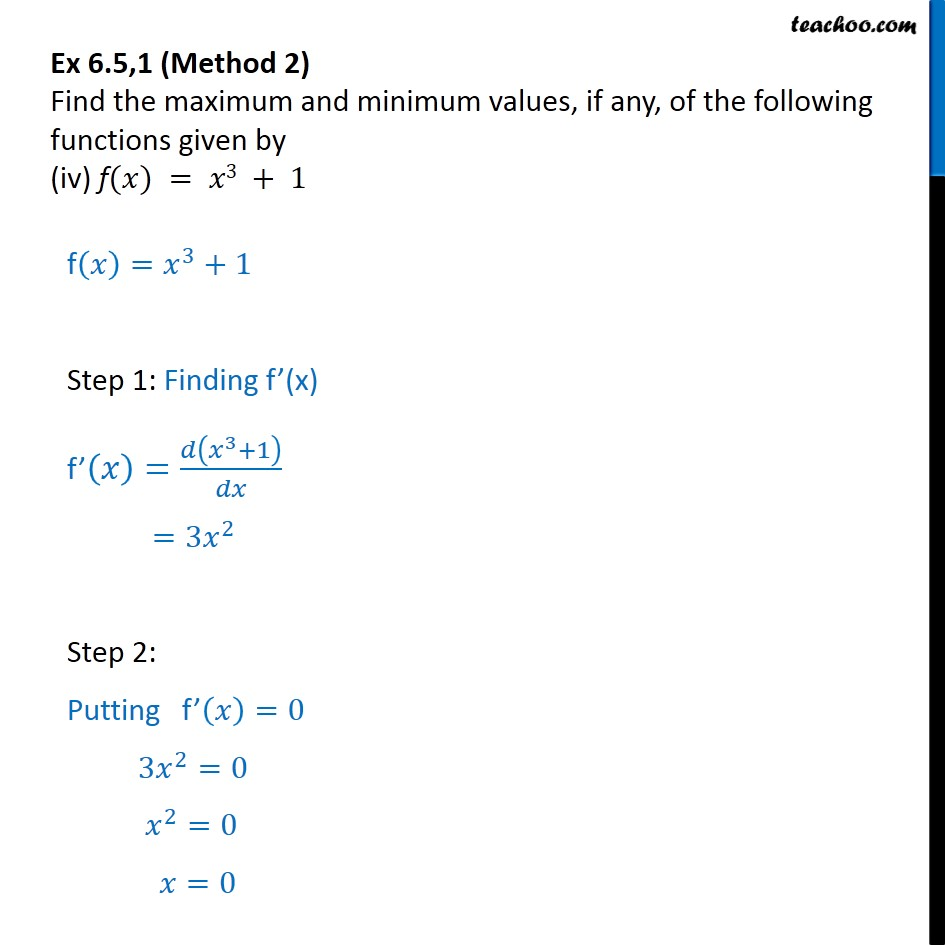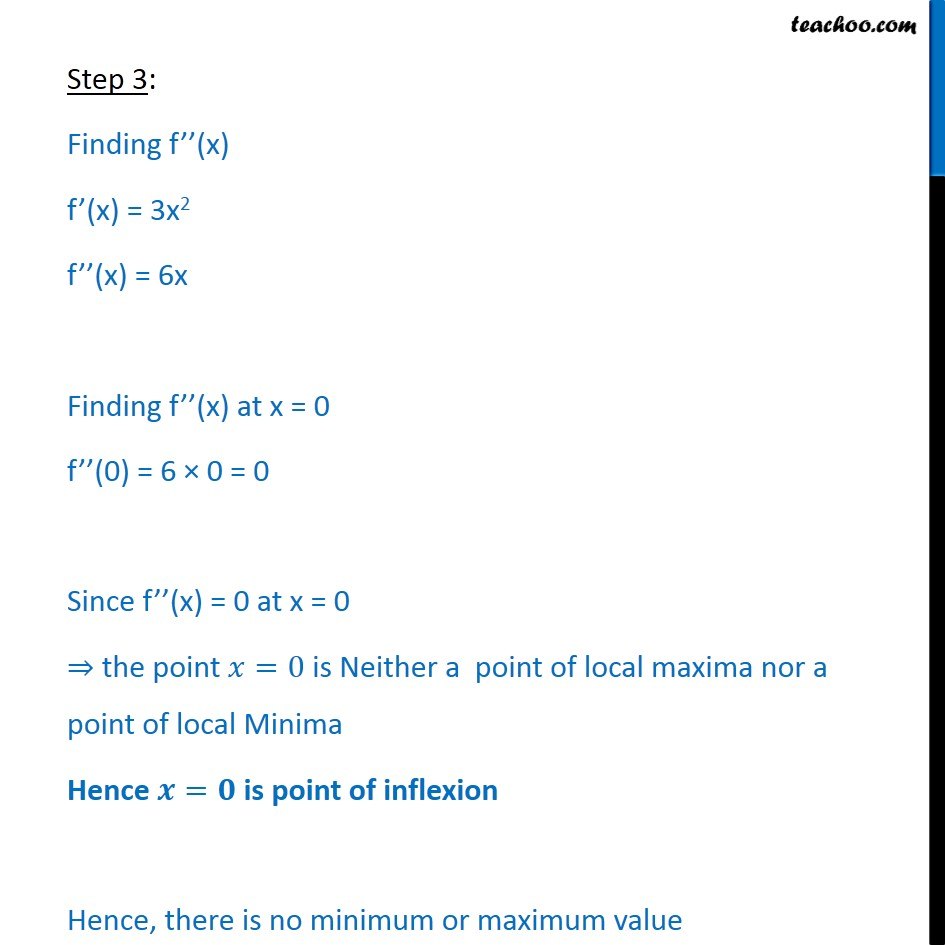1. Chapter 6 Class 12 Application of Derivatives
2. Serial order wise
3. Ex 6.5

Transcript

Ex 6.5,1 (Method 1) Find the maximum and minimum values, if any, of the following functions given by (i) f (𝑥) = (2𝑥 – 1)^2 + 3 f(𝑥)=(2𝑥−1)^2+3 Hence, Minimum value of (2𝑥−1)^2 = 0 Minimum value of (2𝑥−1^2 )+3 = 0 + 3 = 3 Also, there is no maximum value of 𝑥 ∴ There is no maximum value of f(x) Ex 6.5,1 (Method 2) Find the maximum and minimum values, if any, of the following functions given by (i) f (𝑥) = (2𝑥 – 1)^2+3 Step 1: Finding f’(x) f(𝑥)=(2𝑥−1)^2+3 f’(𝑥)= 2(2𝑥−1) Step 2: Putting f’(𝑥)=0 2(2𝑥−1)=0 2𝑥 – 1 = 0 2𝑥 = 1 𝑥 = 1/2 Step 3: Thus, x = 1/2 is the minima Finding minimum value f(𝑥)=(2𝑥−1)^2+3 Putting 𝑥 = 1/2 f(1/2)=(2 × 1/2−1)^2+3= (1−1)^2+3= 3 ∴ Minimum value = 3 There is no maximum value Ex 6.5,1 (Method 3) Find the maximum and minimum values, if any, of the following functions given by (i) 𝑓 (𝑥)= (2𝑥 – 1)^2 + 3 Double Derivative Test f(𝑥)=(2𝑥−1)^2+3 f’(𝑥)=2(2𝑥−1)^(2−1) = 2(2𝑥−1) Putting f’(𝑥)=0 2(2𝑥−1)=0 (2𝑥−1)=0 2𝑥 = 0 + 1 𝑥 = 1/2 Now, finding f’’(𝑥) f’(𝑥)=2(2𝑥−1) f’(𝑥) = 4𝑥 – 2 f’’(𝑥)= 4 So, f’’ (1/2) = 4 Since f’’ (1/2) > 0 , 𝑥 = 1/2 is point of local minima Putting 𝑥 = 1/2 , we can calculate minimum value f(𝑥) = (2𝑥−1)^2+3 f(1/2)= (2(1/2)−1)^2+3= (1−1)^2+3= 3 Hence, Minimum value = 3 There is no Maximum value Ex 6.5,1 (Method 1) Find the maximum and minimum values, if any, of the following functions given by (ii) f (𝑥) = 9𝑥2+12𝑥+2 Step 1: Finding f’(x) f (𝑥)=9𝑥2+12𝑥+2 Diff. w.r.t 𝑥 f’(𝑥)=18𝑥+12 f’(𝑥)=6(3𝑥+2) Step 2: Putting f’(𝑥)=0 6(3𝑥+2)=0 3𝑥+2=0 3𝑥=−2 𝑥=(−2)/( 3) Step 3: Hence 𝑥=(−2)/3 is point of minima of f(𝑥) Finding minimum value of f(𝑥) at 𝑥=(−2)/3 f(𝑥)=9𝑥^2+12𝑥+2 Putting 𝑥=(−2)/3 f(𝑥)=9((−2)/3)^2+12((−2)/3)+2=9(4/3)−12(2/3)+2=−2 Thus, Minimum value of f(𝒙)=−𝟐 There is no maximum value Ex 6.5,1 (Method 2) Find the maximum and minimum values, if any, of the following functions given by (ii) f (𝑥) = 9𝑥2+12𝑥+2 Step 1: f (𝑥)=9𝑥2+12𝑥+2 Diff. w.r.t 𝑥 f’(𝑥)=𝑑(9𝑥^2 + 12𝑥 + 2)/𝑑𝑥 f’(𝑥)=18𝑥+12 f’(𝑥)=6(3𝑥+2) Step 2: Putting f’(𝑥)=0 6(3𝑥+2)=0 3𝑥+2=0 3𝑥=−2 𝑥=(−2)/3 Step 3: Finding f’’(𝑥) f’(𝑥)= 6(3𝑥+2) Again diff w.r.t 𝑥 f’’(𝑥)=𝑑(6(3𝑥+2))/𝑑𝑥 f’’(𝑥)=6 𝑑(3𝑥 + 2)/𝑑𝑥 f’’(𝑥)=6(3+0) f’’(𝑥)=6(3) f’’(𝑥)=18 So, f’’((−2)/3)=18 Since f’’(𝑥)>0 is for 𝑥=(−2)/3 𝑥=(−2)/3 is point of local minima Step 4: Putting 𝑥=(−2)/3 we can calculate minimum value of f(𝑥) f (𝑥)=9𝑥2+12𝑥+2 f ((−2)/3)=9((−2)/3)^2+12((−2)/3)+2 =9(4/9)+12((−2)/3)+2 =4−8+2 =−2 Hence minimum value = –2 There is no maximum value Ex 6.5,1 (Method 1) Find the maximum and minimum values, if any, of the following functions given by (iii) 𝑓 (𝑥) = – (𝑥 – 1)^2+10 f(𝑥)=−(𝑥−1)^2+10 Step 1: Diff w.r.t 𝑥 f’(𝑥)=𝑑(−(𝑥−1)^2+10)/𝑑𝑥 f’(𝑥) = –2(𝑥−1)(𝑑(𝑥−1)/𝑑𝑥)+0 f’(𝑥) = –2(𝑥−1)(1−0) + 0 f’(𝑥)=−2(𝑥−1) Step 2: Putting f’(𝑥)=0 –2(𝑥−1)=0 (𝑥−1)=0 𝑥=1 Step 3: Hence, 𝑥=1 is point of Maxima & No point of Minima Thus, f(𝑥) has maximum value at 𝑥=1 f(𝑥)=−(𝑥−1)^2+10 Putting 𝑥=1 f(1)=−(1−1)^2+10 = 0 + 10 = 10 Maximum value of f(𝑥) is 10 There is no minimum value of f(𝒙) Ex 6.5,1 (Method 2) Find the maximum and minimum values, if any, of the following functions given by (iii) 𝑓 (𝑥) = – (𝑥 – 1)^2+10 f(𝑥)=−(𝑥−1)^2+10 Step 1: Diff w.r.t 𝑥 f’(𝑥)=𝑑(−(𝑥−1)^2+10)/𝑑𝑥 f’(𝑥) = –2(𝑥−1)(𝑑(𝑥−1)/𝑑𝑥)+0 f’(𝑥) = –2(𝑥−1)(1−0) + 0 f’(𝑥)=−2(𝑥−1) Step 2: Putting f’(𝑥)=0 –2(𝑥−1)=0 (𝑥−1)=0 𝑥=1 Step 3: Finding f’’(𝑥) f’(𝑥)=−2(𝑥−1) Again diff w.r.t 𝑥 f’’(𝑥)=𝑑(−2(𝑥 − 1))/𝑑𝑥 =−2 𝑑(𝑥 − 1)/𝑑𝑥 =−2(1−0) =−2 Since f’’(𝑥) < 0 for 𝑥=1 Hence f(𝑥) has Maximum value at 𝑥=1 Finding maximum value of f(𝑥) f(𝑥)=−(𝑥−1)^2+10 Putting 𝑥=1 f(𝑥) =−(1−1)^2+10 = 0 + 10 = 10 Maximum value of f(𝒙) is 10 There is no minimum value of f(𝒙) Ex 6.5,1 (Method 1) Find the maximum and minimum values, if any, of the following functions given by (iv) f(𝑥) = 𝑥3 + 1 f(𝑥)=𝑥^3+1 Step 1: Finding f’(x) f’(𝑥)=𝑑(𝑥^3+1)/𝑑𝑥 =3𝑥^2 Step 2: Putting f’(𝑥)=0 3𝑥^2=0 𝑥^2=0 𝑥=0 Step 3: ⇒ Therefore by first derivate test, the point 𝑥=0 is Neither a point of local maxima nor a point of local Minima Hence 𝒙=𝟎 is point of inflexion Hence, there is no minimum or maximum value Ex 6.5,1 (Method 2) Find the maximum and minimum values, if any, of the following functions given by (iv) f(𝑥) = 𝑥3 + 1 f(𝑥)=𝑥^3+1 Step 1: Finding f’(x) f’(𝑥)=𝑑(𝑥^3+1)/𝑑𝑥 =3𝑥^2 Step 2: Putting f’(𝑥)=0 3𝑥^2=0 𝑥^2=0 𝑥=0 Step 3: Finding f’’(x) f’(x) = 3x2 f’’(x) = 6x Finding f’’(x) at x = 0 f’’(0) = 6 × 0 = 0 Since f’’(x) = 0 at x = 0 ⇒ the point 𝑥=0 is Neither a point of local maxima nor a point of local Minima Hence 𝒙=𝟎 is point of inflexion Hence, there is no minimum or maximum value

Ex 6.5